# Algal (non)-linear mixed model example

Version 2013-01-06 00:04:43

## Preliminaries

Inspired by a question from Diego Pujoni on r-sig-mixed, and (a few days later, presumably because there was no response) on r-help.

The parameters of the problem are that the intercept (0-day value) is known to be identical across groups and individuals.

library(nlme)
library(lme4)
## library(reshape2)
library(plyr)
library(ggplot2)
theme_set(theme_bw())
library(bbmle) ## for AICtab
library(splines) ## for profiles


Data:

d <- data.frame(Day = rep(c(0,2,4,6,8,10),each=9),
Group = rep(c("c","c","c","t1","t1","t1","t2","t2","t2"),6),
Individual = rep(1:9,6),
X = c(0.71,0.72,0.71,0.72,0.72,0.72,0.70,0.69,0.70,0.72,0.72,
0.71,0.72,0.72,0.71,0.71,0.70,0.71,0.73,0.73,0.69,0.74,
0.69,0.73,0.67,0.71,0.69,0.71,0.71,0.72,0.70,0.71,0.70,
0.52,0.64,0.60,0.70,0.73,0.73,0.67,0.66,0.71,0.47,0.56,
0.54,0.65,0.73,0.73,0.67,0.71,0.58,0.44,0.52,0.58))


Just for the heck of it, plot the data both with lattice and with ggplot2.

library(lattice)
xyplot(jitter(X)~Day, groups=Group, data=d,type=c("a","p"),
auto.key=list(space="right"))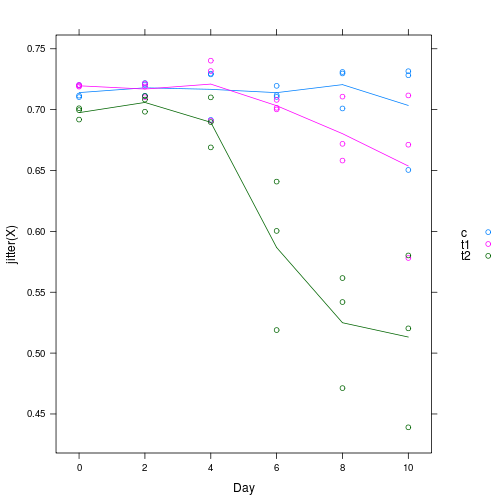ggplot version has two small advantages: 1. Add lines both by individual and group average [should be as easy with stat_summary as with type="a" in xyplot, but there is a bug in the latest ggplot version …]); 2. Adjust point size rather than jittering to visualize overlapping points.

(Both of these would of course be possible with a custom panel.xyplot …)

## have to aggregate by hand: stat_summary bug
d2 <- ddply(d,c("Day","Group"),summarise,X=mean(X))
(g1 <- ggplot(d,aes(x=Day,y=X,colour=Group))+
stat_sum(aes(size=factor(..n..)),alpha=0.5)+
scale_size_discrete(range=c(2,5),name="npts")+
geom_line(aes(group=Individual),alpha=0.5)+
geom_line(data=d2,lwd=2,alpha=0.8)+
scale_colour_brewer(palette="Dark2"))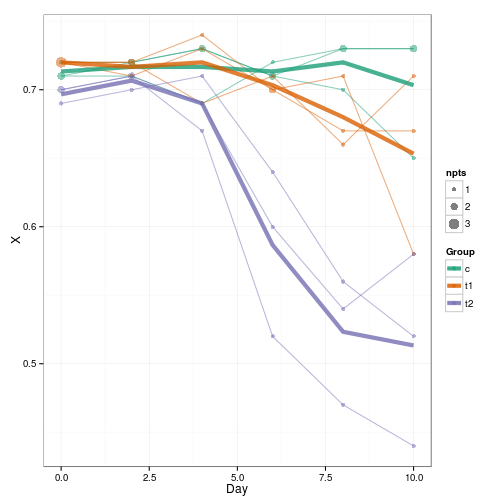The main conclusions from these pictures are that (1) we probably ought to be using a nonlinear rather than a linear model; (2) there might be some heteroscedasticity (larger variance at lower means, as though there is a “ceiling” to the data at $$\approx X=0.7$$); it does look as though there could be among-individual variation (based especially on the t2 data, where the individual curves are approximately parallel). However, we'll also try linear fits for illustration (even though they won't be very good):

## Using nlme

Linear fits with lme fail:

LME <- lme(X ~ 1, random = ~Day|Individual, data=d)

## Error: nlminb problem, convergence error code = 1 message = iteration
## limit reached without convergence (10)


If we run this with control=lmeControl(msVerbose=TRUE))) we get a lot of output, ending with:

47:    -65.306481:  5.38940 0.705107  179.050
48:    -65.306489:  5.42212 0.705107  184.783
49:    -65.306493:  5.45375 0.705106  190.516
50:    -65.306499:  5.47352 0.705104  194.382
50:    -65.306499:  5.47352 0.705104  194.382


Unsurprisingly, a more complex model allowing for Group*Day effects fails too:

LME1 <- lme(X ~ Group*Day, random = ~Day|Individual, data=d)

## Error: nlminb problem, convergence error code = 1 message = iteration
## limit reached without convergence (10)


I tried to fit a non-linear model using SSfpl, a self-starting four-parameter logistic model (with parameters left-asymptote, right-asymptote, midpoint, scale parameter). This works fine for an nls fit, giving reasonable results:

nlsfit1 <- nls(X ~ SSfpl(Day, asymp.L, asymp.R, xmid, scale),data=d)
coef(nlsfit1)

## asymp.L asymp.R    xmid   scale
##  0.7138  0.6248  6.0999  0.9863


Can use gnls to fit group-level differences (although for some reason I need to specify starting values, even though the help file would lead me to believe I don't have to … perhaps I do when params is specified?)

My first attempt is apparently a little too ambitious for gnls:

svec <-  list(asymp.L=0.7,
asymp.R=c(0.6,0,0),
xmid=c(5,0,0),
scale=c(1,0,0))
gnlsfit1 <- gnls(
X ~ SSfpl(Day, asymp.L, asymp.R, xmid, scale),
data=d,
params=list(asymp.R+scale+xmid~Group,asymp.L~1),
start=svec)

## Error: step halving factor reduced below minimum in NLS step


But I can succeed if I allow only asymp.R to vary among groups:

svec2 <-  list(asymp.L=0.7,
asymp.R=c(0.6,0,0),
xmid=6,
scale=1)
gnlsfit2 <- gnls(X ~ SSfpl(Day, asymp.L, asymp.R, xmid, scale),data=d,
params=list(asymp.R~Group,asymp.L++scale+xmid~1),
start=svec2)


Plot predicted values:

predframe <- with(d,expand.grid(Day=unique(Day),Group=levels(Group)))
predframe$X <- predict(gnlsfit2,newdata=predframe) g1 + geom_line(data=predframe,linetype=3,lwd=2,alpha=0.8)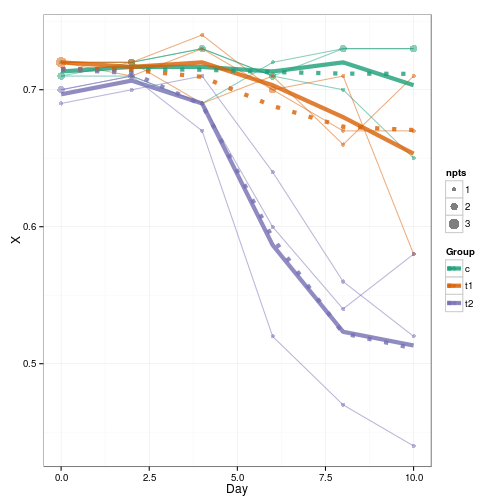These look pretty good (it would be nice to get confidence intervals too, but that's a little bit too much of a pain for right now – need to use either delta method or bootstrapping). dp <- data.frame(d,res=resid(gnlsfit2),fitted=fitted(gnlsfit2)) (diagplot1 <- ggplot(dp,aes(x=factor(Individual), y=res,colour=Group))+ geom_boxplot(outlier.colour=NULL)+ scale_colour_brewer(palette="Dark2"))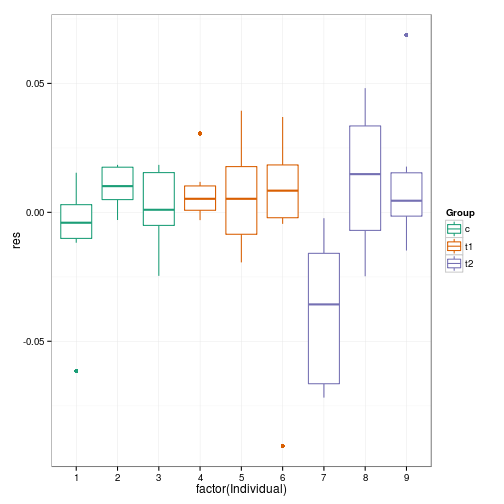With the exception of individual #7 there's not a lot of evidence for among-individual variation … if we wanted an excuse to ignore among-individual variation we could use anova(lm(res~Individual,data=dp))  ## Analysis of Variance Table ## ## Response: res ## Df Sum Sq Mean Sq F value Pr(>F) ## Individual 1 0.0000 0.000012 0.01 0.91 ## Residuals 52 0.0444 0.000854  (whatever philosophical issues this raises about using a large $$p$$-value to accept the null hypothesis that among-individual variation is absent …) More general diagnostic plot – residual vs. fitted, with points from the same individual connected with lines. There is some hint of decreasing variance with increasing mean. (diagplot2 <- ggplot(dp,aes(x=fitted,y=res,colour=Group))+geom_point()+ geom_smooth(aes(group=1),colour="black",method="loess")+ geom_path(aes(group=Individual))+ geom_hline(yintercept=0,lty=2))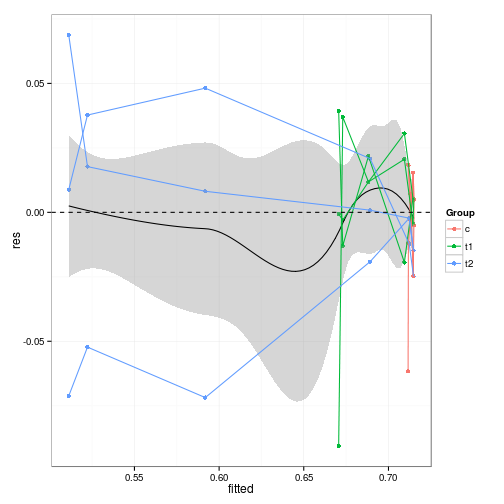I can't use nlme with the more ambitious (three parameters varying by group) model, but I can if I only allow asymp.R to vary: nlmefit1 <- nlme(model = X ~ SSfpl(Day, asymp.L, asymp.R, xmid, scale), fixed = list(asymp.R ~ Group, xmid+scale+asymp.L ~1), random = asymp.R ~ 1 | Individual, start = list(fixed=with(svec2,c(asymp.R,xmid,scale,asymp.L))), data=d)  The estimate of the variance in the right-hand asymptote is non-zero (yay): VarCorr(nlmefit1)  ## Individual = pdLogChol(list(asymp.R ~ 1)) ## Variance StdDev ## 0.0009292 0.03048 ## Residual 0.0004687 0.02165  Adding the random effects doesn't change the parameters much at all: print(mm1 <- merge(data.frame(cc=coef(gnlsfit2)), data.frame(cc=fixef(nlmefit1)),by="row.names"), digits=4)  ## Row.names cc.x cc.y ## 1 asymp.L 0.71508 0.71514 ## 2 asymp.R.Groupt1 -0.04125 -0.04081 ## 3 asymp.R.Groupt2 -0.20162 -0.19986 ## 4 asymp.R.(Intercept) 0.71158 0.71162 ## 5 scale 0.85397 0.82750 ## 6 xmid 5.65084 5.59617  maxdiff <- max(apply(mm1[,-1],1,function(x) abs(diff(x)/mean(x))))  The biggest proportional difference is 3.1% (in the scale parameter). nlmefit2 <- update(nlmefit1,fixed = list(asymp.R+xmid+scale+asymp.L ~1), start = list(fixed=with(svec2,c(asymp.R,xmid,scale,asymp.L))))  We can compare the models via AIC or likelihood ratio test (AICtab from the bbmle package is not essential, but gives pretty output): AICtab(nlmefit1,nlmefit2,weights=TRUE)  ## dAIC df weight ## nlmefit1 0.0 8 0.999 ## nlmefit2 14.2 6 <0.001  anova(nlmefit1,nlmefit2)  ## Model df AIC BIC logLik Test L.Ratio p-value ## nlmefit1 1 8 -229.3 -213.4 122.6 ## nlmefit2 2 6 -215.1 -203.1 113.5 1 vs 2 18.22 1e-04  It would be nice to do an $$F$$ test rather than LRT (i.e. accounting for the finite-size correction), but it's a little bit more work (and probably not really necessary since the answer is so strong). The devdiff <- -2*c(logLik(nlmefit2)-logLik(nlmefit1)) pchisq(devdiff,df=2,lower.tail=FALSE)  ## ## 0.0001106  ## F-test with very large denominator: pf(devdiff/2,df1=2,df2=1000000,lower.tail=FALSE)  ## ## 0.0001106  ## Value Std.Error DF t.value Pr(|t|>1) ## asymp.R.(Intercept) 0.7116 0.0206 40.0000 34.47 < 2e-16 *** ## asymp.R.Groupt1 -0.0408 0.0292 40.0000 -1.40 0.16990 ## asymp.R.Groupt2 -0.1999 0.0301 40.0000 -6.65 5.8e-08 *** ## xmid 5.5962 0.2382 40.0000 23.49 < 2e-16 *** ## scale 0.8275 0.2175 40.0000 3.81 0.00048 *** ## asymp.L 0.7151 0.0051 40.0000 140.31 < 2e-16 *** ## --- ## Signif. codes: 0 '***' 0.001 '**' 0.01 '*' 0.05 '.' 0.1 ' ' 1  We don't really know the relevant denominator df, but the summary above suggests the denominator df is 40 (based on the usual type of classical-block-design analogy for df counting, see Pinheiro and Bates 2000 or the glmm wikidot faq). pf(devdiff/2,df1=2,df2=40,lower.tail=FALSE)  ## ## 0.0005493  ## nlmer With help from Reinhold Kliegl (see below) I think I can now get the correct model specification for nlmer, but I can't find a convenient syntax for non-trivial fixed effect modeling (i.e. in this case allowing some of the parameters to vary by group) – and when I do construct the correct syntax, nlmer seems too fragile to get the answer. This StackOverflow question asks the same question (syntax, not overcoming fragility). The basic RE model (without group effects) works fine: nlmerfit1 <- nlmer( X ~ SSfpl(Day, asymp.L, asymp.R, xmid, scale) ~ asymp.R|Individual, start = list(nlpars=c(asymp.L=0.7, asymp.R=0.6,xmid=5,scale=1)),data=d)  With help from Reinhold Kliegl I got a bit farther (only to be frustrated again: see below). As I understand it, one simply has to construct one's own function that encapsulates the fixed-effect structure; to be used with nlmer, the function also needs to compute the gradient with respect to the fixed-effect parameters. This is a bit of a pain, but can be slightly automated with some hackery by modifying the function generated by the deriv function: 1. Construct dummy variables: mm <- model.matrix(~Group,data=d) grp2 <- mm[,2] grp3 <- mm[,3] ## or just grp2=as.numeric(d$Group)==2 etc.

1. Construct a function to evaluate the predicted value and its gradient; the grouping structure is hard-coded:
nparams <- c("A",paste0("B",0:2),"xmid","scale")
fpl <- deriv(~A+((B0+B1*grp2+B2*grp3)-A)/(1+exp((x-xmid)/scale)),
nparams,
function.arg=c("x",nparams))
attr(fpl,"pnames") <- nparams

1. Hack the resulting function by inserting lines to match the parameter names passed to the function, and assign those parameter names to the gradient matrix:
tmpstr <- deparse(fpl)
L1 <- grep("^ +\\.value +<-",tmpstr)
L2 <- grep("^ +attr\$$\\.value",tmpstr) tmpstr2 <- c(tmpstr[1:L1], paste0(".actualArgs <- as.list(match.call()[", deparse(nparams),"])"), tmpstr[(L1+1):(L2-1)], "dimnames(.grad) <- list(NULL, .actualArgs)", tmpstr[L2:length(tmpstr)]) fpl <- eval(parse(text=tmpstr2))  Attempt the fit: nlmerfit2 <- nlmer( X ~ fpl(Day, asymp.L, asymp.R1, asymp.R2, asymp.R3, xmid, scale) ~ asymp.R1|Individual, start = list(nlpars=c(asymp.L=0.7, asymp.R1=0.6,asymp.R2=0,asymp.R3=0,xmid=5,scale=1)),data=d)  ## Error: step factor reduced below 0.001 without reducing pwrss  Fails (but I think this is due to the fragility of nlmer rather than anything fundamentally wrong with the setup). To be sure I should generate a larger, artificial data set along the same lines and see if I can get it to work. Right now we are using the development version of lme4, i.e. version 0.99999911.0. We can get an answer (but not a sensible answer) with the stable (lme4.0) version. detach("package:lme4",unload=TRUE) library("lme4.0")  ## Attaching package: 'lme4.0'  ## The following object is masked from 'package:nlme': ## ## lmList, VarCorr  packageVersion("lme4.0")  ##  '0.999999.1'  nlmerfit2 <- nlmer( X ~ fpl(Day, asymp.L, asymp.R1, asymp.R2, asymp.R3, xmid, scale) ~ asymp.R1|Individual, start = c(asymp.L=0.7, asymp.R1=0.6,asymp.R2=0,asymp.R3=0,xmid=5,scale=1),data=d)  The results are silly! fixef(nlmerfit2)  ## asymp.L asymp.R1 asymp.R2 asymp.R3 xmid scale ## -156.16916 45.81031 -0.01931 -0.12157 4428.96516 3548.54685  range(predict(nlmerfit2))  ##  -156.2 4429.0  I can't be sure (short of getting the Word of God from DB) that there is no easier way to do fixed effects in nlmer, but I have stared at the source code and spent several hours struggling with various permutations to do this in a more “built-in” way, without success. ## AD Model Builder We can also use AD Model Builder to tackle this problem. It seems (see below) that it can handle the more complex models, such as fitting group effects of more of the parameters; to my surprise, some of these models turn out to be informative (I expected that we were more or less at the limits of the signal in the data). Partly due to my lower level of familiarity with ADMB, this was a bit of a slog, and I only succeeded in the end by building up via gradual steps. ### Minimal example First try it without random effects, grouping variables, etc. (i.e. equivalent to nls fit above). setup_admb()  ##  "/usr/local/admb"  admb_model0 <- " PARAMETER_SECTION vector pred(1,nobs) // predicted values PROCEDURE_SECTION pred = asympL+(asympR-asympL)/(1.0+exp(-(Day-xmid)/scale)); f = regression(X,pred); " writeLines(admb_model0,con="algae0.tpl")  ## set up data: adjust names, etc. d0 <- subset(d,select=c(Day,X)) d0 <- c(list(nobs=nrow(d)),as.list(d0)) ## starting values: adjust names, add values svec3 <- svec2 names(svec3) <- gsub("\\.","",names(svec3)) ## remove dots svec3asympR <- 0.6 ## single value ## run admbfit0 <- do_admb("algae0", data=d0, params=svec3, run.opts=run.control(checkparam="write", checkdata="write"))  Works fine: ## admb nls ## asympL 0.713782 0.713782 ## asympR 0.624793 0.624793 ## xmid 6.099903 6.099905 ## scale 0.986272 0.986270  ### Fixed effects model Now try with fixed effects grouping (equivalent to gnlsfit2 above), using the dummy variables constructed above (could also use if statements, or a vector of R values in a for loop with R[Group[i]], or (best choice) pass a model matrix for R …). We have to use elem_div tather than / for elementwise division of two vectors. admb_model1 <- " PARAMETER_SECTION vector pred(1,nobs) // predicted values vector Rval(1,nobs) // PROCEDURE_SECTION Rval = asympR1+asympR2*grp2+asympR3*grp3; pred = asympL+elem_div(Rval-asympL,1.0+exp(-(Day-xmid)/scale)); f = regression(X,pred); " writeLines(admb_model1,con="algae1.tpl")  d1 <- c(d0,list(grp2=grp2,grp3=grp3)) svec4 <- c(rename(svec3,c(asympR="asympR1")), list(asympR2=0,asympR3=0)) admbfit1 <- do_admb("algae1", data=d1, params=svec4, run.opts=run.control(checkparam="write", checkdata="write"))  Trying it with a model matrix instead: admb_model1B <- " PARAMETER_SECTION vector pred(1,nobs) // predicted values vector Rval(1,nobs) // PROCEDURE_SECTION Rval = XR*Rvec; pred = asympL+elem_div(Rval-asympL,1.0+exp(-(Day-xmid)/scale)); f = regression(X,pred); " writeLines(admb_model1B,con="algae1B.tpl")  d1B <- c(d0,list(XR=mm)) svec4B <- rename(svec3,c(asympR="Rvec")) svec4BRvec <- c(0.6,0,0) admbfit1B <- do_admb("algae1B", data=d1B, params=svec4B, run.opts=run.control(checkparam="write", checkdata="write"))  Works, with identical parameters (except for order/names), of course. ### Random effects Now adding the random effects. The regression function is not fully implemented for random effects models (although this should be fixed in an upcoming version), so we subtitute the formula \( n/2 \log(\text{RSS}/n)$$, where RSS is the residual sum of squares (coded as norm2(X-pred)):

admb_model2 <- "
PARAMETER_SECTION
vector pred(1,nobs)       // predicted values
vector Rval(1,nobs)       //

PROCEDURE_SECTION
Rval = XR*Rvec+Rsigma*(Z*Ru);
pred = asympL+elem_div(Rval-asympL,1.0+exp(-(Day-xmid)/scale));
f = 0.5*nobs*log(norm2(X-pred)/nobs)+norm2(Ru);
"
d2 <- c(d0,list(XR=mm,Z=model.matrix(~factor(Individual)-1,data=d)))
svec5 <- c(svec4B,list(Rsigma=list(0.1,bounds=c(0,10))))
data=d2,
params=svec5,
re=list(Ru=max(d$Individual)), run.opts=run.control(checkparam="write", checkdata="write"))  ##  TRUE  Since ADMB doesn't handle sparse matrices, and doesn't penalize looping, it would be slightly more efficient to implement the random effects as for (i=1; i<=nobs; i++) Rval[i] += Rsigma*Ru[Group[i]], but I'm being lazy/I like the compactness and extensibility of the matrix representation … Now we can finally test for fixed-effect differences in parameters other than R: admb_model3 <- " PARAMETER_SECTION vector pred(1,nobs) // predicted values vector Rval(1,nobs) // vector scaleval(1,nobs) vector xmidval(1,nobs) sdreport_vector opred(1,nobs) // output predictions PROCEDURE_SECTION Rval = XR*Rvec+Rsigma*(Z*Ru); scaleval = Xscale*scalevec; xmidval = Xxmid*xmidvec; pred = asympL+elem_div(Rval-asympL, 1.0+exp(-elem_div(Day-xmidval,scaleval))); opred=pred; f = 0.5*nobs*log(norm2(X-pred)/nobs)+norm2(Ru); " writeLines(admb_model3,con="algae3.tpl") d3 <- c(d2,list(Xscale=mm,Xxmid=mm)) svec6 <- rename(svec5,c(xmid="xmidvec",scale="scalevec")) svec6$xmidvec <- c(svec6$xmidvec,0,0) svec6$scalevec <- c(svec6$scalevec,0,0) admbfit3 <- do_admb("algae3", data=d3, params=svec6, re=list(Ru=max(d$Individual)),
run.opts=run.control(checkparam="write",
checkdata="write"))


Results:

AICtab(admbfit0,admbfit1,admbfit2,admbfit3,weights=TRUE)

##          dAIC  df weight
## admbfit1  34.9 6  < 0.001
## admbfit0 111.3 4  < 0.001

summary(admbfit3)

## Model file: algae3_gen
## Negative log-likelihood:  -214.2      AIC:  -450.5
## Coefficients:
##            Estimate Std. Error z value Pr(>|z|)
## asympL      0.71222    0.00341  208.62  < 2e-16 ***
## Rvec.1      0.70342    0.03399   20.69  < 2e-16 ***
## Rvec.2     -0.06823    0.06525   -1.05   0.2957
## Rvec.3     -0.18892    0.04592   -4.11  3.9e-05 ***
## xmidvec.1   9.31528    1.59892    5.83  5.7e-09 ***
## xmidvec.2  -0.27981    1.56654   -0.18   0.8582
## xmidvec.3  -3.79499    1.60802   -2.36   0.0183 *
## scalevec.1  0.96047    0.70150    1.37   0.1709
## scalevec.2 -0.31566    0.87108   -0.36   0.7171
## scalevec.3 -0.15714    0.71820   -0.22   0.8268
## Rsigma      0.07297    0.02290    3.19   0.0014 **
## ---
## Signif. codes:  0 '***' 0.001 '**' 0.01 '*' 0.05 '.' 0.1 ' ' 1


There's a very large AIC difference. Likelihood ratio/$$F$$ tests as shown above for the nlme fits are left as an exercise …

For the plot, it's nice to redo the fit with the parameters specified per-group, rather than as baseline+contrast:

d4 <- d3
d4$XR <- d4$Xscale <- d4$Xxmid <- model.matrix(~Group-1,data=d) svec7 <- svec6 for (i in c("Rvec","xmidvec","scalevec")) svec7[[i]] <- rep (svec7[[i]],3) admbfit3B <- do_admb("algae3", data=d4, params=svec7, re=list(Ru=max(d$Individual)),
run.opts=run.control(checkparam="write",
checkdata="write"
))

library(coefplot2)
cc <- coeftab(admbfit3B)[1:11,] ## omit RE parameters
coefplot2(list(cc),intercept=TRUE)Now we're annoyed about the scaling, so (with some fussing) we can replot the group-varying parameters in separate panels: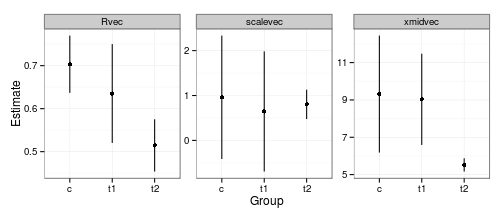Diagnostic plots:

## drop conditional mode/individual-R estimates
admb3res <- admb3pred-d$X dp2 <- data.frame(d,res=admb3res,fitted=admb3pred) diagplot1 %+% dp2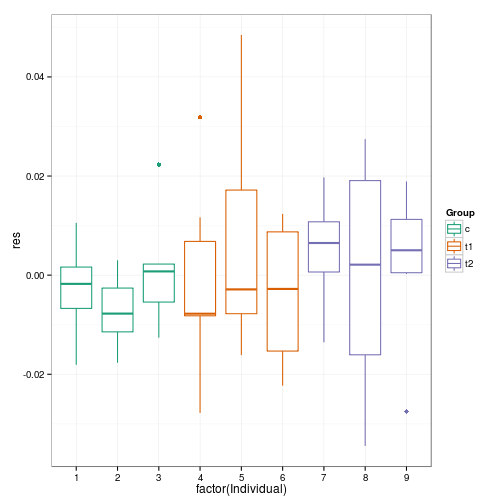Perhaps a hint of greater variance in the two treatment groups? Fitted vs. residual: diagplot2 %+% dp2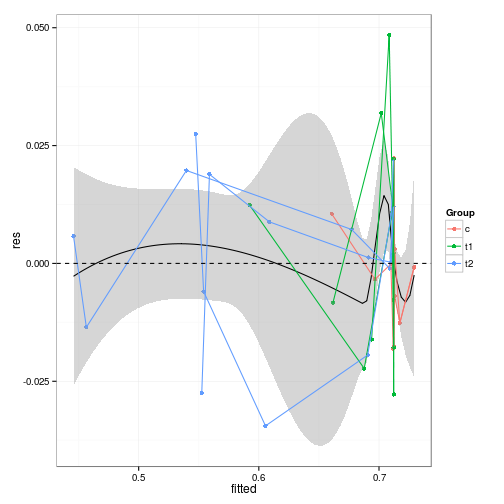Overlay predictions (dashed lines): g1 + geom_line(data=dp2,aes(y=fitted,group=Individual),lty=2)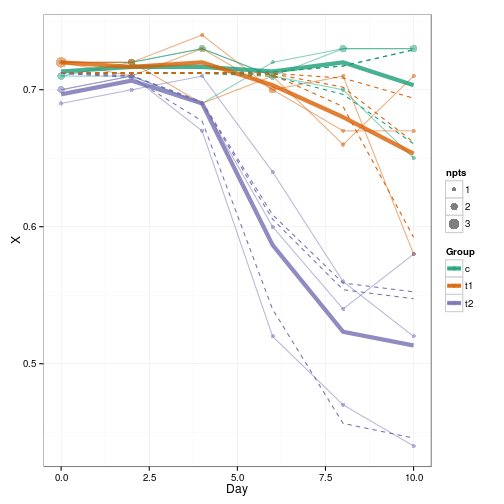It would be nice but a bit more tedious to generate smooth prediction curves (i.e. for intermediate Day values). ## Conclusions • The main conclusion from the parameter estimates is that the third group drops off a little bit earlier (xmidvec is smaller) as well as dropping farther (Rvec is lower). ## Likelihood profiling Computing a likelihood profile for $$\sigma^2_R$$ is not too hard to code (now that I've implemented phase support in R2admb, which allows us to fix the values of selected parameters by making their phases negative), but is a bit of a pain to run: it's slow, and the computation craps out with non-positive-definite matrices at several points right around the lower confidence bound; I ran another set of values to try to cover the region adequately (grubby details hidden). svec8 <- svec6 Rsigmavec <- seq(0,0.24,by=0.01) fitfun <- function(Rsigma) { ## cat(".") svec8$Rsigma <- list(Rsigma,phase=-1)
data=d3,
params=svec8,
re=list(Ru=max(d\$Individual)),
run.opts=run.control(checkparam="write",
checkdata="write")),silent=TRUE)
}
## try to fill in holes
Rsigmavec2 <- seq(0.032,0.048,by=0.002)


The profiled points with the interpolation spline and the likelihood ratio test cutoff line: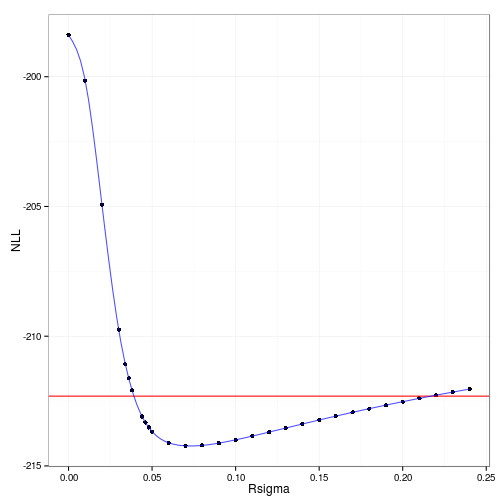The 95% profile confidence intervals on $$\sigma^2_R$$ (derived from inverting an interpolation spline fitted to these points) are {0.0386,0.2169}.

I haven't computed it, but the transformed profile plot (on a $$y$$ scale corresponding to the square-root deviation of the deviance from the minimum deviance, so that a quadratic profile would be a symmetric V) shows that the quadratic approximation would be fairly bad for this case …

ggplot(na.omit(proftab12),aes(Rsigma,sqrt(2*(NLL-min(NLL,na.rm=TRUE)))))+
geom_point()+labs(x=~{sigma^2}[R],y=~sqrt(Delta*"deviance"))+
geom_hline(yintercept=1.96,colour="red")+
geom_line(data=sdat,colour="blue",alpha=0.7)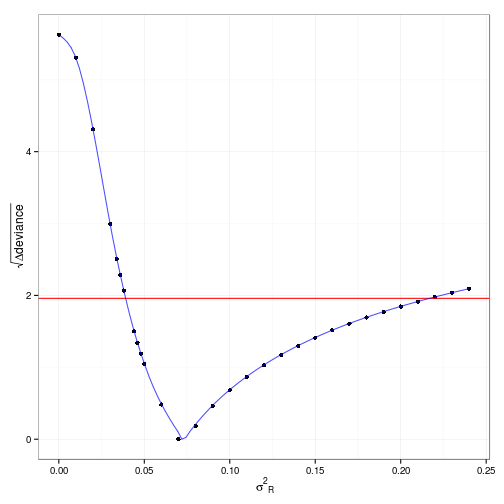## To do / extensions

• Discuss denominator df issues more. Parametric bootstrap/MCMC?
• One could try to put random effects in the xmid and scale parameters as well, although I think that would be a little ambitious for this data set.
• There might be additional patterns in the variance (either residual or among-individual) among groups or as a function of X.